# Pythagoras Theorem: Formula, Proof, Examples And Applications

## Pythagoras Theorem

Pythagoras theorem: The Pythagoras theorem is an important concept used while solving questions in Mathematics. The theorem explains the relation between the sides of a triangle. The Pythagoras theorem was derived by Pythagoras, a Greek philosopher of the sixth century B.C who declared it as an essential property of the right-angled triangles. The property is, hence, named after him. If any triangle holds the Pythagoras property, then it is certainly a right-angled triangle. Let’s take a look at the statement, formulas, proof, applications and examples of the Pythagoras theorem.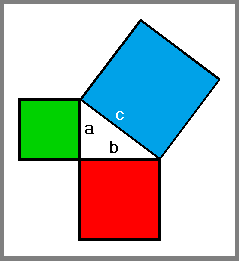### Pythagoras theorem: Statement & Formula

In a right-angled triangle, the side opposite to the right angle is called the hypotenuse and the other two sides are known as the legs of the right-angled triangle. The hypotenuse is the longest side and the other 2 sides are named as Perpendicular and base.

Statement of Pythagoras theorem: The Pythagoras theorem states that “In a right triangle, the square of the hypotenuse is equal to the sum of the squares of the other two sides”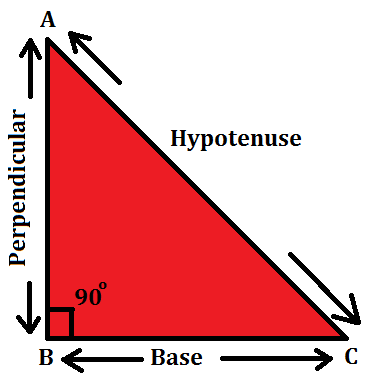From the figure given above, the Pythagoras theorem formula can be derived as:

### Pythagoras Theorem: Proof

We are given a right triangle ABC right angled at B. Let BD be the perpendicular to the hypotenuse AC. You must know the theorem “If a perpendicular is drawn from the vertex of the right angle of a right triangle to the hypotenuse then triangles on both sides of the perpendicular are similar to the whole triangle and to each other”

Let us draw BD ⊥ AC (See Fig)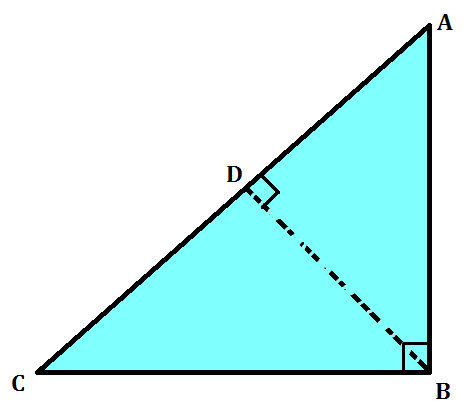∠ A = ∠ A
and ∠ ADB = ∠ ABC
So, ∆ ADB ~ ∆ ABC
Similarly, ∆ BDC ~ ∆ ABC
Now, ∆ ADB ~ ∆ ABC

So, AD/AB = AB/AC (Sides are proportional)

or, AD. AC = AB²  (1)
Also, ∆ BDC ~ ∆ ABC

So, CD/BC = BC/AC or CD . AC = BC²   (2)

AD. AC + CD . AC = AB² + BC²
or, AC (AD + CD) = AB² + BC²
or, AC. AC = AB² + BC²

or, AC² = AB² + BC²

### Pythagoras theorem: ApplicationsThe applications of Pythagoras theorem can be as follows:

• To solve questions based on triangles and to find if the triangle is a right-angled triangle or not.
• To calculate the diagonal of a square.
• This theorem is used in architecture, woodworking, and other physical construction projects

### Pythagoras theorem: Triplets

You must know the Pythagoras triplets to apply this theorem to various concepts. Some of the Pythagoras triplets are given below:

• 3, 4, 5
• 5, 12, 13
• 7, 24, 25
• 8, 15, 17
• 9, 40, 41
• 11, 60, 61
• 12, 35, 37
• 20, 21, 29

### Pythagoras theorem: Examples and Questions

Example 1: ∆ ABC is right-angled at C. If AC = 5 cm and BC = 12 cm find the length of AB.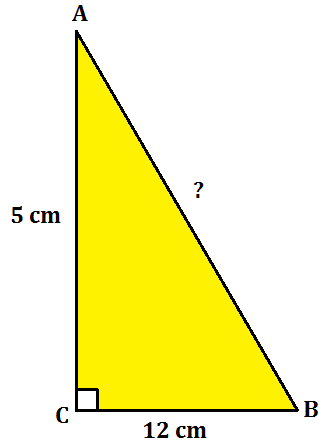Refer the figure given on the right.

As the triangle is right-angled, by Pythagoras theorem,
AB² = AC² + BC²

AB² = 5² + 12²
AB² = 25 + 144
AB² = 169 = 13²

Hence, AB = 13 cm

Example 2: A ladder is placed against a wall such that its foot is at a distance of 2.5 m from the wall and its top reaches a window 6 m above the ground. Find the length of the ladder.

Let AB be the ladder and CA be the wall with the window at A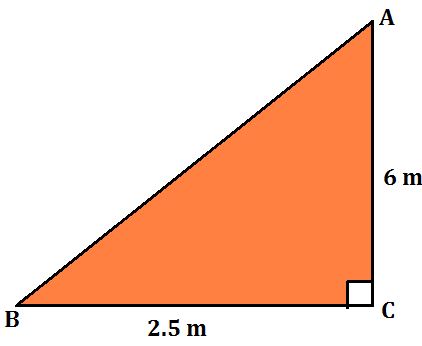Also, BC = 2.5 m and CA = 6 m

From Pythagoras Theorem, we have:
AB² = AC² + BC²
AB² = 6² + (2.5)² = 42.25

So, AB = 6.5
Thus, the length of the ladder is 6.5 m.

Example 3: Determine whether the triangle whose lengths of sides are 3 cm, 4 cm, 5 cm is a right-angled triangle.

Solution: 3² = 9, 4² = 16 and 5² = 25

We find, 3² + 4² = 5²

Therefore, the triangle is right-angled.

Example 4: The side of a square is given to be 4 cm. Find the length of the diagonal of a square.

Solution: We need to find the length of the diagonal AC. As we know, all sides of a square are equal and each angle is of 90 degrees, ADC is a right-angled triangle.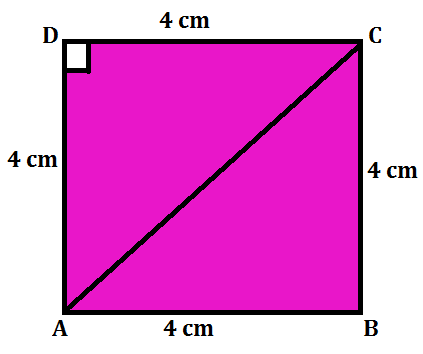By Pythagoras theoram,

AC² = 4² + 4² = 16 + 16

AC² = 32, So, AC= 4√2

The diagonal of the square is 4√2 cm.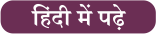### 21 Days | 21 Free All India Mocks Challenge, Practice from Home

June Current Affairs PDF

×

Thank You, Your details have been submitted we will get back to you.Join India's largest learning destination

What You Will get ?

•Job Alerts
•Daily Quizzes
•Subject-Wise Quizzes
•Current Affairs
•Previous year question papers
•Doubt Solving session

ORJoin India's largest learning destination

What You Will get ?

•Job Alerts
•Daily Quizzes
•Subject-Wise Quizzes
•Current Affairs
•Previous year question papers
•Doubt Solving session

ORJoin India's largest learning destination

What You Will get ?

•Job Alerts
•Daily Quizzes
•Subject-Wise Quizzes
•Current Affairs
•Previous year question papers
•Doubt Solving session

Enter the email address associated with your account, and we'll email you an OTP to verify it's you.Join India's largest learning destination

What You Will get ?

•Job Alerts
•Daily Quizzes
•Subject-Wise Quizzes
•Current Affairs
•Previous year question papers
•Doubt Solving session

Enter OTP

Please enter the OTP sent to
/6

Did not recive OTP?

Resend in 60sJoin India's largest learning destination

What You Will get ?

•Job Alerts
•Daily Quizzes
•Subject-Wise Quizzes
•Current Affairs
•Previous year question papers
•Doubt Solving sessionJoin India's largest learning destination

What You Will get ?

•Job Alerts
•Daily Quizzes
•Subject-Wise Quizzes
•Current Affairs
•Previous year question papers
•Doubt Solving session

Almost there

+91Join India's largest learning destination

What You Will get ?

•Job Alerts
•Daily Quizzes
•Subject-Wise Quizzes
•Current Affairs
•Previous year question papers
•Doubt Solving session

Enter OTP

Please enter the OTP sent to Edit Number

Did not recive OTP?

Resend 60

By skipping this step you will not recieve any free content avalaible on adda247, also you will miss onto notification and job alerts

Are you sure you want to skip this step?

By skipping this step you will not recieve any free content avalaible on adda247, also you will miss onto notification and job alerts

Are you sure you want to skip this step?